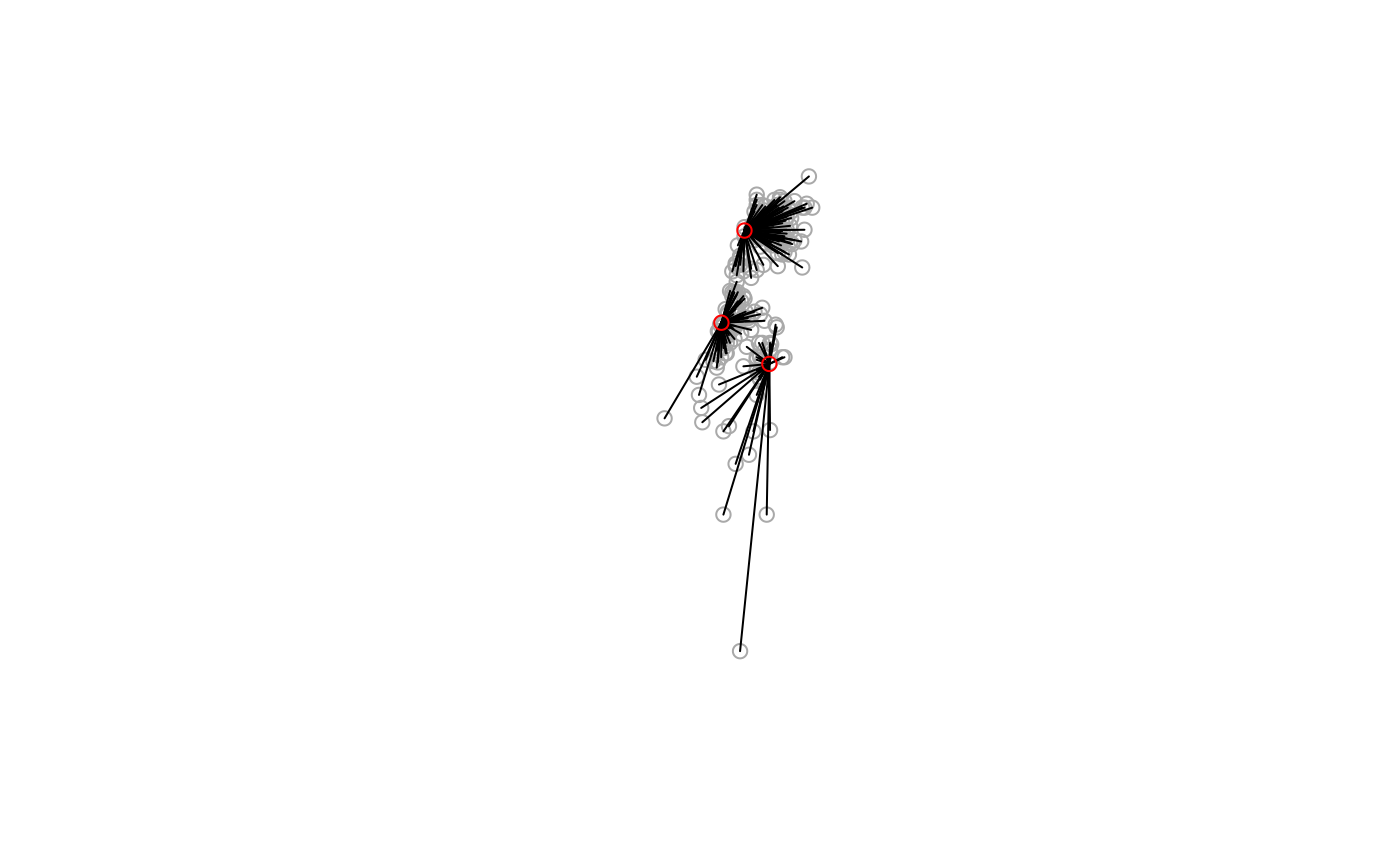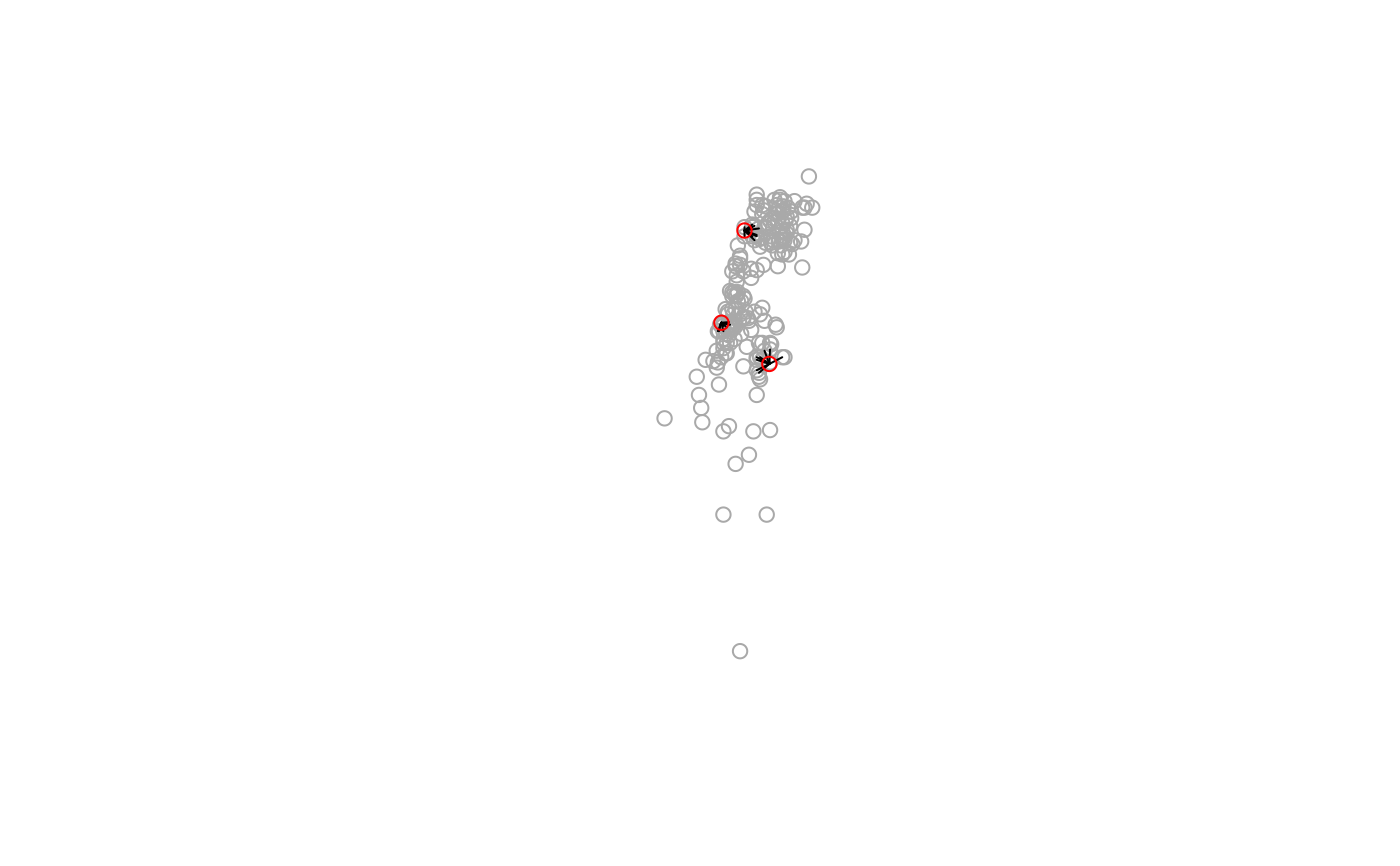Returns a line layer with line segments which connect the nearest feature(s) from y for each feature in x. This is mostly useful for graphical purposes (see Note and Examples below).

st_connect(x, y, ids = NULL, progress = TRUE, ...)

## Arguments

x Object of class sf or sfc Object of class sf or sfc A sparse list representation of features to connect such as returned by function st_nn. If NULL the function automatically calculates ids using st_nn Display progress bar? (default TRUE) Other arguments passed to st_nn when calculating ids, such as k and maxdist

## Value

Object of class sfc with geometry type LINESTRING

## Note

The segments are straight lines, i.e., they correspond to shortest path assuming planar geometry regardless of CRS. Therefore, the lines should serve as a graphical indication of features that are nearest to each other; the exact shortest path between features should be calculated by other means, such as geosphere::greatCircle.

## Examples

# Nearest 'city' per 'town'
l = st_connect(towns, cities, progress = FALSE)
#> lon-lat pointsplot(st_geometry(towns), col = "darkgrey")
plot(st_geometry(l), add = TRUE)
plot(st_geometry(cities), col = "red", add = TRUE)# Ten nearest 'towns' per 'city'
l = st_connect(cities, towns, k = 10, progress = FALSE)
#> lon-lat pointsplot(st_geometry(towns), col = "darkgrey")
plot(st_geometry(l), add = TRUE)
plot(st_geometry(cities), col = "red", add = TRUE)if (FALSE) {

# Nearest 'city' per 'town', search radius of 30 km
cities = st_transform(cities, 32636)
towns = st_transform(towns, 32636)
l = st_connect(cities, towns, k = nrow(towns), maxdist = 30000, progress = FALSE)
plot(st_geometry(towns), col = "darkgrey")
plot(st_geometry(l), add = TRUE)
plot(st_buffer(st_geometry(cities), units::set_units(30, km)), border = "red", add = TRUE)

# The 20-nearest towns for each water body
water = st_transform(water, 32636)
l = st_connect(water[-1, ], towns, k = 20, dist = 100, progress = FALSE)
plot(st_geometry(water[-1, ]), col = "lightblue", border = NA)
plot(st_geometry(towns), col = "darkgrey", add = TRUE)
plot(st_geometry(l), col = "red", add = TRUE)

# The 2-nearest water bodies for each town
l = st_connect(towns, water[-1, ], k = 2, dist = 100)
plot(st_geometry(water[-1, ]), col = "lightblue", border = NA)
plot(st_geometry(towns), col = "darkgrey", add = TRUE)
plot(st_geometry(l), col = "red", add = TRUE)

}